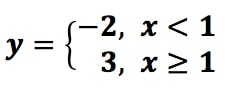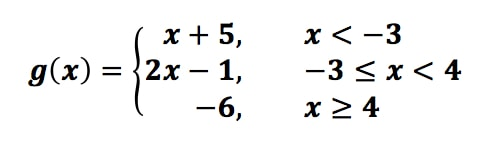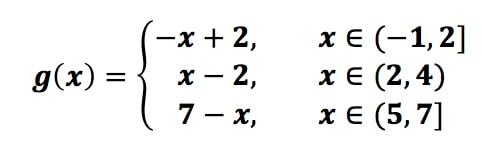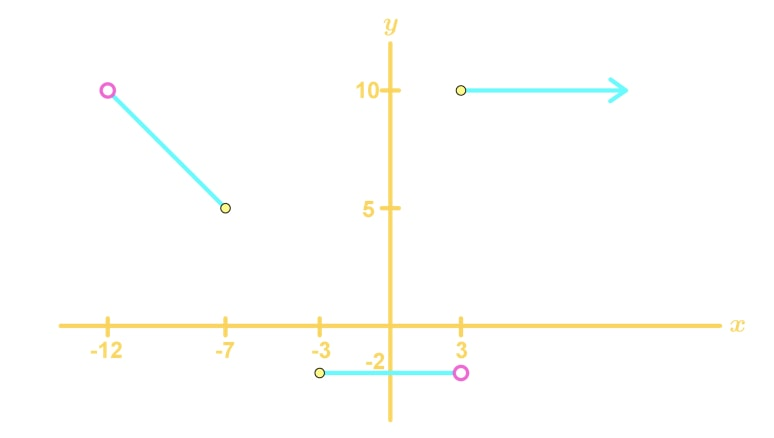# Graphing piecewise linear functions

### Graphing piecewise linear functions

#### Lessons

• 1.
For the following functiona)
Sketch the graph

b)
State the domain

c)
State the range

• 2.
For the following functiona)
Sketch the graph

b)
State the domain

c)
State the range

• 3.
For the following functiona)
Sketch the graph

b)
State the domain

c)
State the range

• 4.
Write a piecewise function that models the following graph:• 5.
a)
Graph$f(x)$ = |3$x$ - 2|; and

b)
Express$f(x)$ = |3$x$ - 2| as a piecewise function